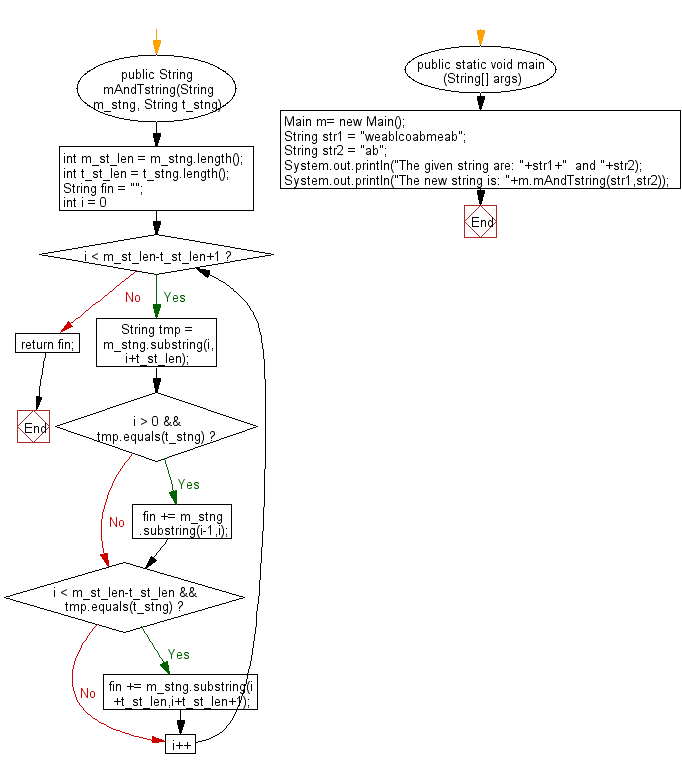﻿ Java exercises: New string with each character of just before and after of a non-empty substring whichever it appears in a non-empty given string - w3resource# Java String Exercises: New string with each character of just before and after of a non-empty substring whichever it appears in a non-empty given string

## Java String: Exercise-85 with Solution

Write a Java program to make a new string with each character of just before and after of a non-empty substring whichever it appears in a non-empty given string.

Sample Solution:

Java Code:

``````import java.util.*;
public class Main
{
public String mAndTstring(String m_stng, String t_stng)
{
int m_st_len = m_stng.length();
int t_st_len = t_stng.length();
String fin = "";
for (int i = 0; i < m_st_len-t_st_len+1; i++)
{
String tmp = m_stng.substring(i,i+t_st_len);
if (i > 0 && tmp.equals(t_stng))
fin += m_stng.substring(i-1,i);
if (i < m_st_len-t_st_len && tmp.equals(t_stng))
fin += m_stng.substring(i+t_st_len,i+t_st_len+1);
}
return fin;
}

public static void main (String[] args)
{
Main m= new Main();
String str1 =  "weablcoabmeab";
String str2 =  "ab";
System.out.println("The given string are: "+str1+"  and "+str2);
System.out.println("The new string is: "+m.mAndTstring(str1,str2));
}
}
``````

Sample Output:

```The given string are: weablcoabmeab  and ab
The new string is: elome
```

Flowchart:Java Code Editor:

Improve this sample solution and post your code through Disqus

What is the difficulty level of this exercise?

﻿

## Java: Tips of the Day

Parsing dates:

```import java.io.*;
import java.util.*;
import java.text.*;

String s = "2001/09/23 14:39";

SimpleDateFormat formatter = new SimpleDateFormat ("yyyy/MM/dd H:mm");
Date d = formatter.parse(s, new ParsePosition(0));
```